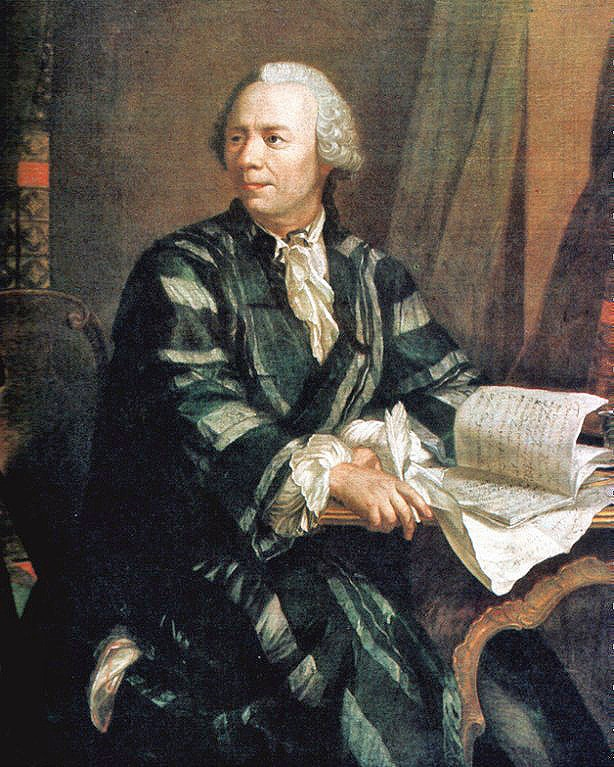# The Most Beautiful EquationHaving noticed the name of this article, many of you have already guessed what it’s going to be about. Also, here are additional hints: this short but quite famous formula may be seen on the T-shirts or cups worldwide; mathematicians and physicists name it “the greatest equation” in some scientific polls. Actually, we’re talking about the Euler’s identity, named to honor the great Swiss mathematician Leonhard Euler. So what exactly is great about this formula?The sense of beauty and elegance of identity comes from a combination of five most important numbers of mathematical constants: $e$ – base of the natural logarithm, $i$ – the square root of $-1, \pi, 1$ and $0$. Looking closely at it, most people pay attention to the exponent: what does it mean to raise the number to the imaginary degree? Patience, we’ll get to that! To explain the origin of this identity, we should firstly get a general formula, found by Euler, and then show that equality is only a special case of this formula. The general formula is unique by itself and has a lot of great applications in math, physics and engineering.

The first step in our explanation is to understand that most of the functions in math can be represented as an infinite sum in powers of the argument. Here is an example:

$\sin{x}=x- \frac{x^3}{3!}+ \frac{x^5}{5!}- \frac{x^7}{7!}+…$

Here $x$ is measured in radians, not in degrees. We can get a good approximation of $\sin{x}$ for a particular value of $x$, using only the first few terms of the series. It is an example of the Taylor series, and it’s pretty easy to deduce this formula using mathematical analysis. Here, we do not demand the knowledge of mathematical analysis, so we just ask you to take our word for it.

Corresponding formula for the cosine is:

$\cos{x}=1- \frac{x^2}{2!}+ \frac{x^4}{4!}- \frac{x^6}{6!}+…$

And finally, formula for the exponent is:

$e^x=1+x+\frac{x^2}{2!}+ \frac{x^3}{3!}+\frac{x^4}{4!}+…$Number $e$ is the constant, equaled to $2,71828 \ldots$, and Euler was the first person to recognize its fundamental importance in mathematics, and made up the last formula (the initial two were found by Isaac Newton). There are even books, devoted to the number e (for example, Maor, E. (1994). E, the story of a number).

Around 1740, Eiler looked at these three formulas located almost the same way as you see them here. You can also see that each term in the third equation also appears in any of the preceding ones. However, half of the members in the first equality are negative, while each member of the latter is positive. Most people have left these representations in their original form, but Euler had found the law in them. He was the first who added the first two formulas: $\sin{x}+\cos{x}=1+x-\frac{x^2}{2!}-\frac{x^3}{3!}+\frac{x^4}{4!}+\frac{x^5}{5!}-\frac{x^6}{6!}-\frac{x^7}{7!}+…$ (1)

Pay attention to the sequence of signs in this series: + + – – + + – – + + …, it is repeated in groups of $4$ signs. Euler noticed that the same sequence of characters happens when we raise imaginary unit $i$ in the integer degree:

$i^0=+1, i^1=+i, i^2=-1, i^3=-i, i^4=+1, i^5=+i,…$

It means that we can replace $x$ in the last formula to $xi$ and get:

$e^{xi}=1+xi-\frac{x^2}{2!}-\frac{ix^3}{3!}+\frac{x^4}{4!}+\frac{ix^5}{5!}-\frac{x^6}{6!}-\frac{ix^7}{7!}+…$ (2)

Now, signs in $(2)$ correspond to the signs from $(1)$ formula, and a new series coincides with the previous one, except that the expansion terms of $\sin{x}$ are multiplied by $i$. So, we get exactly:

$e^{xi}=\cos{x}+i \sin{x}$It is an amazing and mysterious result, which indicates the existence of a close connection between the number $e$, $\sin$ and $\cos$ in trigonometry. Although $e$ is known only from the examples related neither to the geometry nor to triangles. Besides its elegance and strangeness, it would be difficult to overestimate the importance of this formula in mathematics, which even increased since its discovery. It appears everywhere, and it was recently published a book of about 400 pages (Nahin P. Dr. Euler’s Fabulous Formula, 2006), devoted to a description of some of its applications.

Now, going back to the magic equation, let’s substitute any real number $x$ in it, and get a complex number. One possible option  is $x = \pi$. Recall from trigonometry that $\pi$ radians is $180$ degrees. The cosine of $180$ degrees is equal to $1$, and the sine of the same angle is $0$.
Therefore,

$e^{i \pi}=-1+i \cdot 0$, or $e^{i \pi}=-1$

In conclusion, we may dare and say that with no exaggeration, math world is full of many beautiful and amazing things, which we are always ready to share with you.

0 Shares
Filed under Math.